Скачать презентацию Surplus Measures 1 An Alternative View of

a499a43ea9b5721508a1dcb10f5a9839.ppt

• Количество слайдов: 17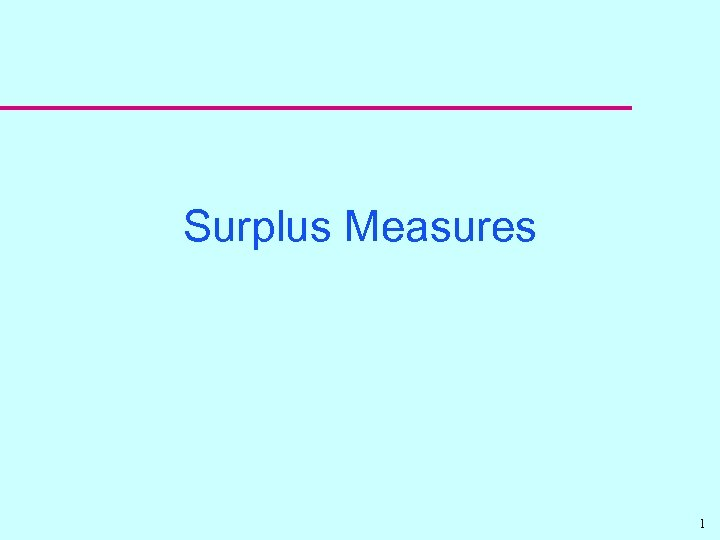Surplus Measures 1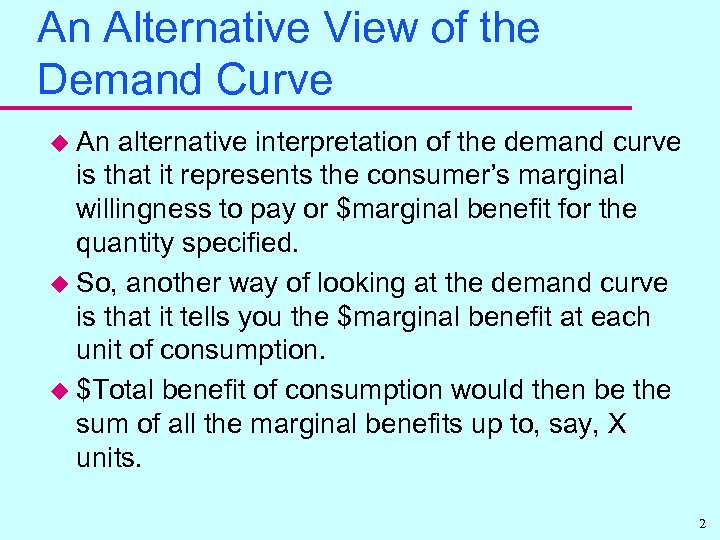An Alternative View of the Demand Curve u An alternative interpretation of the demand curve is that it represents the consumer’s marginal willingness to pay or \$marginal benefit for the quantity specified. u So, another way of looking at the demand curve is that it tells you the \$marginal benefit at each unit of consumption. u \$Total benefit of consumption would then be the sum of all the marginal benefits up to, say, X units. 2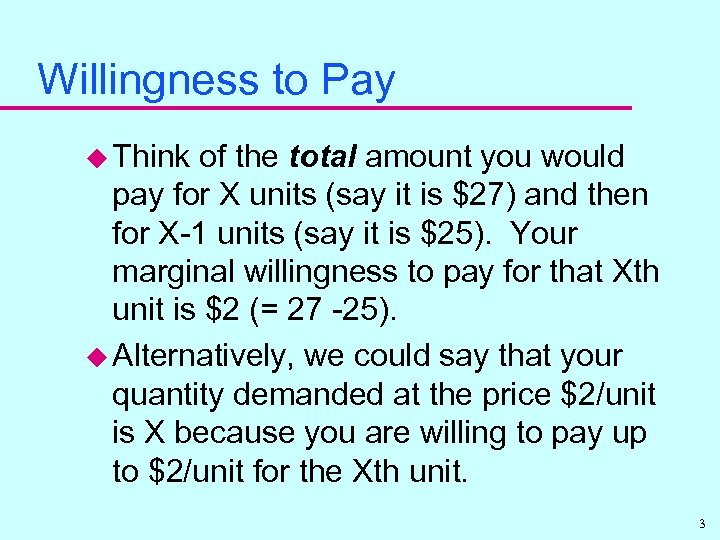Willingness to Pay u Think of the total amount you would pay for X units (say it is \$27) and then for X-1 units (say it is \$25). Your marginal willingness to pay for that Xth unit is \$2 (= 27 -25). u Alternatively, we could say that your quantity demanded at the price \$2/unit is X because you are willing to pay up to \$2/unit for the Xth unit. 3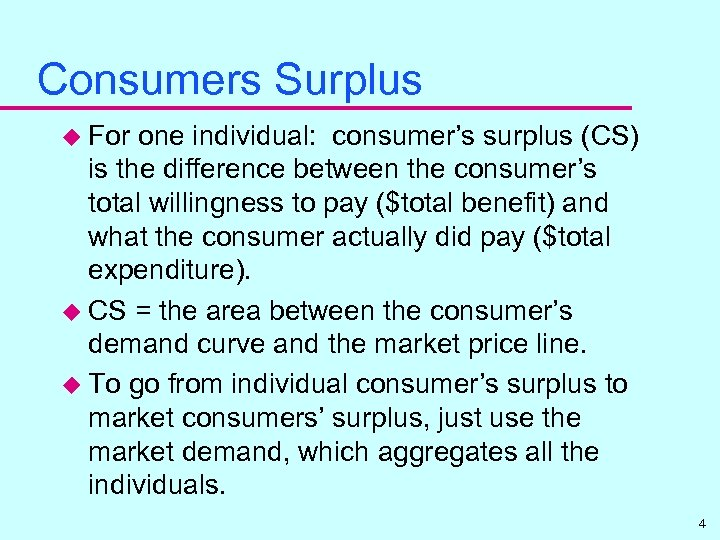Consumers Surplus u For one individual: consumer’s surplus (CS) is the difference between the consumer’s total willingness to pay (\$total benefit) and what the consumer actually did pay (\$total expenditure). u CS = the area between the consumer’s demand curve and the market price line. u To go from individual consumer’s surplus to market consumers’ surplus, just use the market demand, which aggregates all the individuals. 4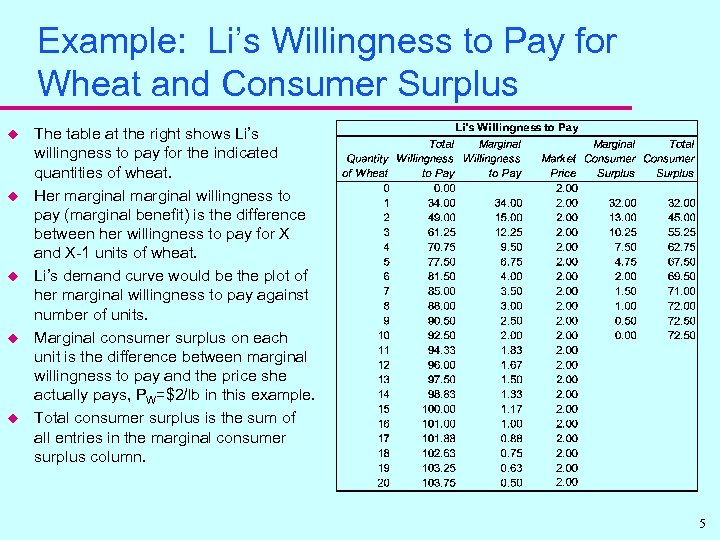Example: Li’s Willingness to Pay for Wheat and Consumer Surplus u u u The table at the right shows Li’s willingness to pay for the indicated quantities of wheat. Her marginal willingness to pay (marginal benefit) is the difference between her willingness to pay for X and X-1 units of wheat. Li’s demand curve would be the plot of her marginal willingness to pay against number of units. Marginal consumer surplus on each unit is the difference between marginal willingness to pay and the price she actually pays, PW=\$2/lb in this example. Total consumer surplus is the sum of all entries in the marginal consumer surplus column. 5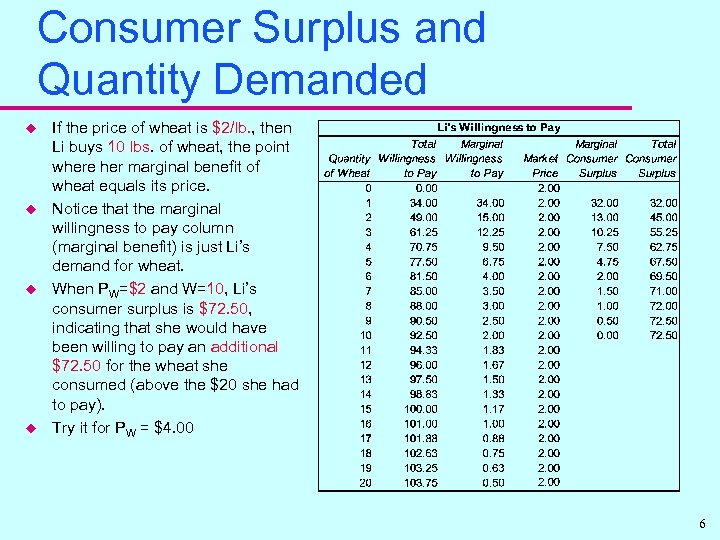Consumer Surplus and Quantity Demanded u u If the price of wheat is \$2/lb. , then Li buys 10 lbs. of wheat, the point where her marginal benefit of wheat equals its price. Notice that the marginal willingness to pay column (marginal benefit) is just Li’s demand for wheat. When PW=\$2 and W=10, Li’s consumer surplus is \$72. 50, indicating that she would have been willing to pay an additional \$72. 50 for the wheat she consumed (above the \$20 she had to pay). Try it for PW = \$4. 00 6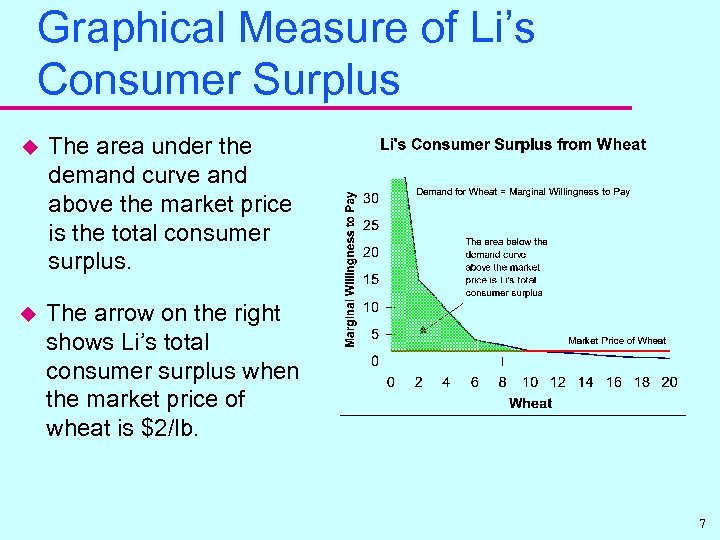Graphical Measure of Li’s Consumer Surplus u The area under the demand curve and above the market price is the total consumer surplus. u The arrow on the right shows Li’s total consumer surplus when the market price of wheat is \$2/lb. 7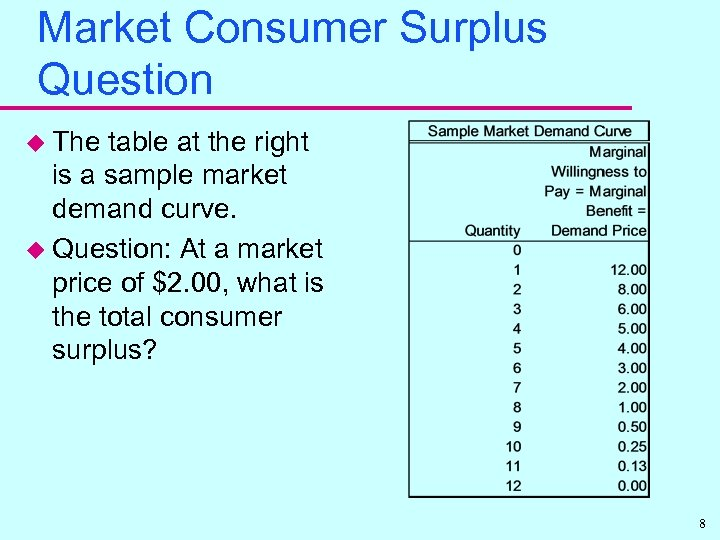Market Consumer Surplus Question u The table at the right is a sample market demand curve. u Question: At a market price of \$2. 00, what is the total consumer surplus? 8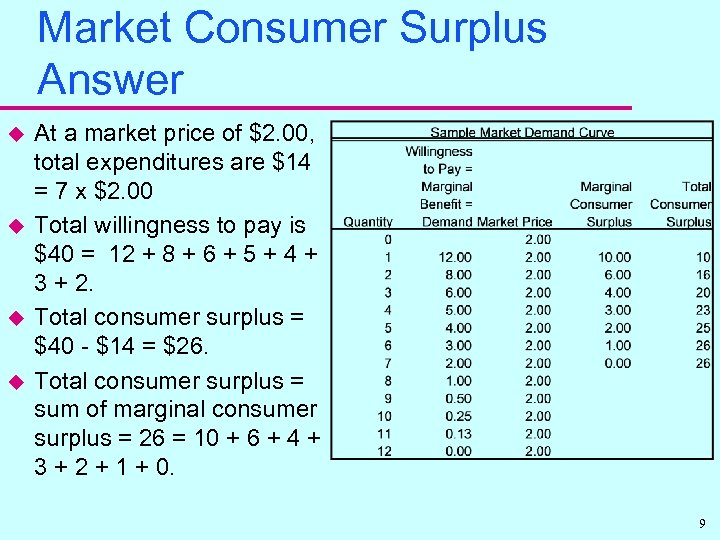Market Consumer Surplus Answer u u At a market price of \$2. 00, total expenditures are \$14 = 7 x \$2. 00 Total willingness to pay is \$40 = 12 + 8 + 6 + 5 + 4 + 3 + 2. Total consumer surplus = \$40 - \$14 = \$26. Total consumer surplus = sum of marginal consumer surplus = 26 = 10 + 6 + 4 + 3 + 2 + 1 + 0. 9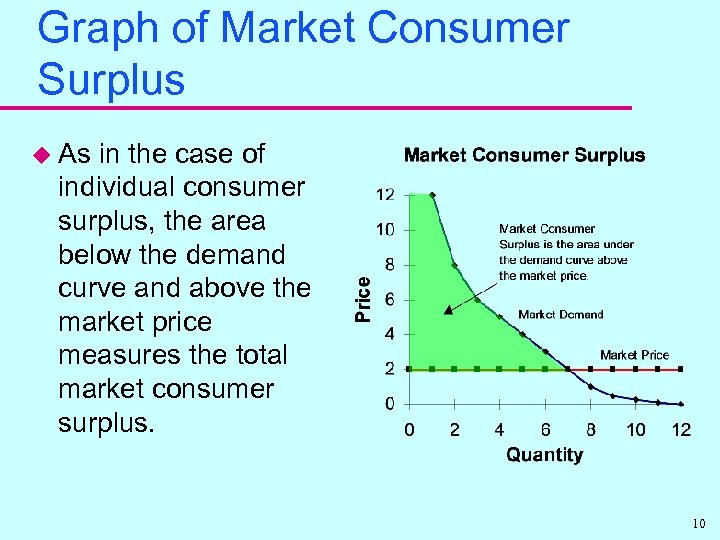Graph of Market Consumer Surplus u As in the case of individual consumer surplus, the area below the demand curve and above the market price measures the total market consumer surplus. 10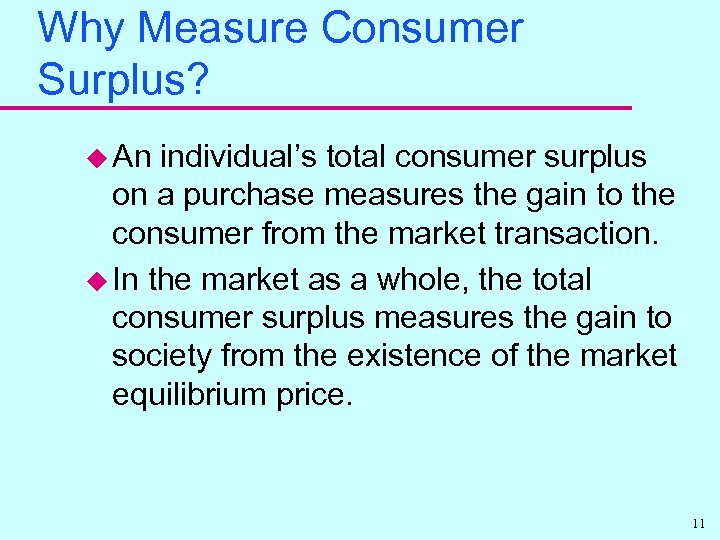Why Measure Consumer Surplus? u An individual’s total consumer surplus on a purchase measures the gain to the consumer from the market transaction. u In the market as a whole, the total consumer surplus measures the gain to society from the existence of the market equilibrium price. 11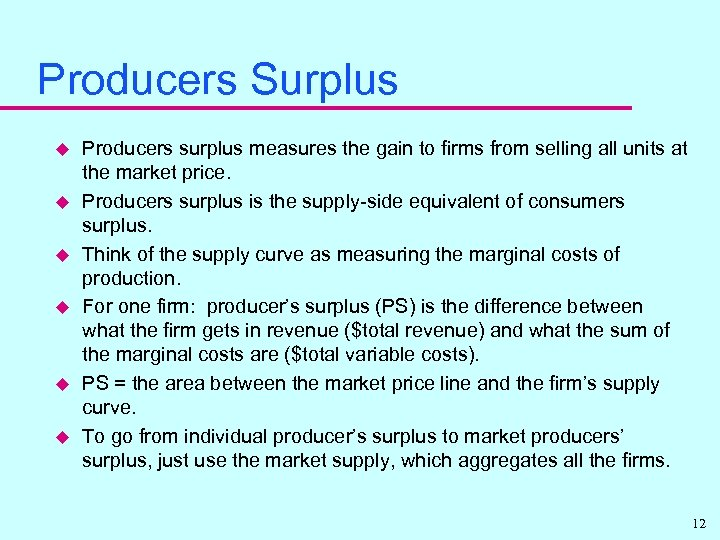Producers Surplus u u u Producers surplus measures the gain to firms from selling all units at the market price. Producers surplus is the supply-side equivalent of consumers surplus. Think of the supply curve as measuring the marginal costs of production. For one firm: producer’s surplus (PS) is the difference between what the firm gets in revenue (\$total revenue) and what the sum of the marginal costs are (\$total variable costs). PS = the area between the market price line and the firm’s supply curve. To go from individual producer’s surplus to market producers’ surplus, just use the market supply, which aggregates all the firms. 12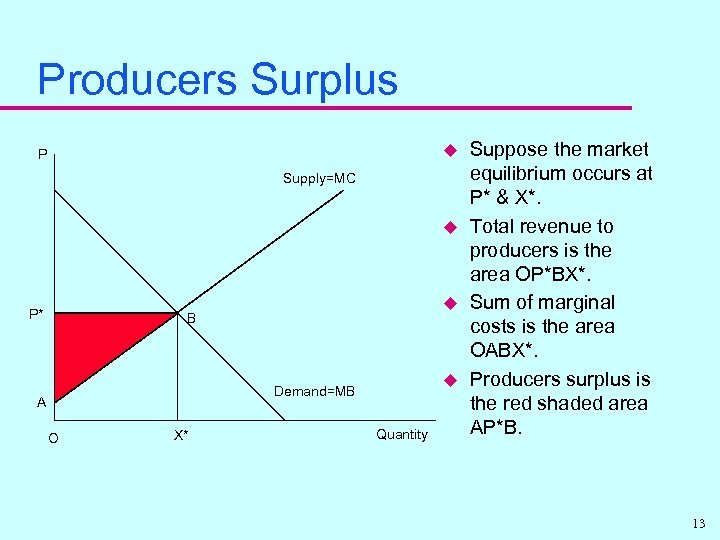Producers Surplus u P Supply=MC u P* u B u Demand=MB A O X* Quantity Suppose the market equilibrium occurs at P* & X*. Total revenue to producers is the area OP*BX*. Sum of marginal costs is the area OABX*. Producers surplus is the red shaded area AP*B. 13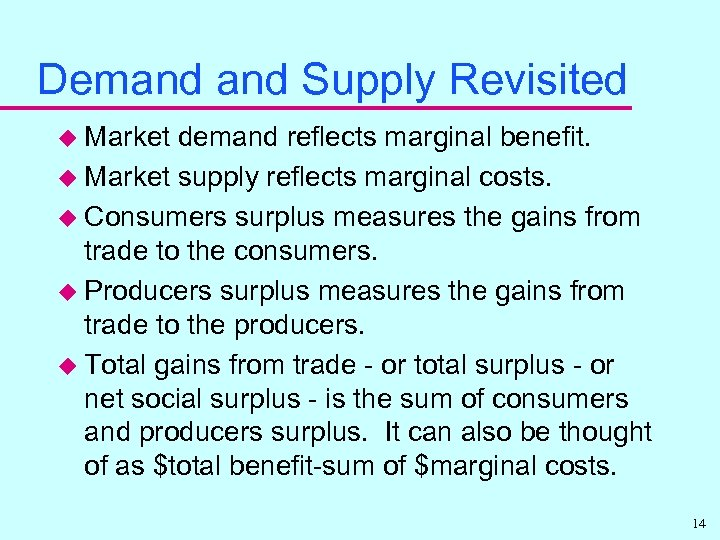Demand Supply Revisited u Market demand reflects marginal benefit. u Market supply reflects marginal costs. u Consumers surplus measures the gains from trade to the consumers. u Producers surplus measures the gains from trade to the producers. u Total gains from trade - or total surplus - or net social surplus - is the sum of consumers and producers surplus. It can also be thought of as \$total benefit-sum of \$marginal costs. 14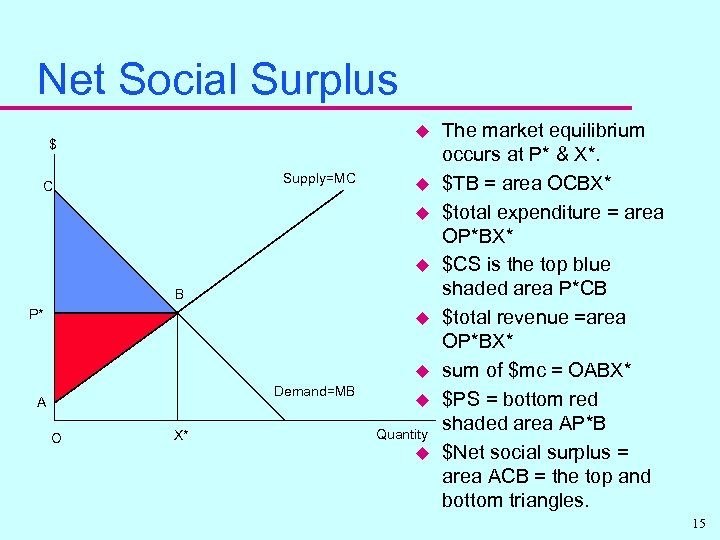Net Social Surplus u \$ Supply=MC C u u u B P* u u Demand=MB A O X* u Quantity u The market equilibrium occurs at P* & X*. \$TB = area OCBX* \$total expenditure = area OP*BX* \$CS is the top blue shaded area P*CB \$total revenue =area OP*BX* sum of \$mc = OABX* \$PS = bottom red shaded area AP*B \$Net social surplus = area ACB = the top and bottom triangles. 15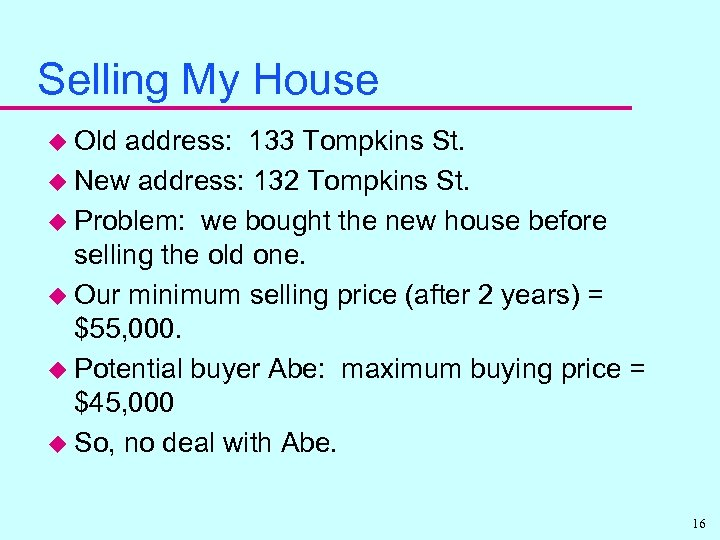Selling My House u Old address: 133 Tompkins St. u New address: 132 Tompkins St. u Problem: we bought the new house before selling the old one. u Our minimum selling price (after 2 years) = \$55, 000. u Potential buyer Abe: maximum buying price = \$45, 000 u So, no deal with Abe. 16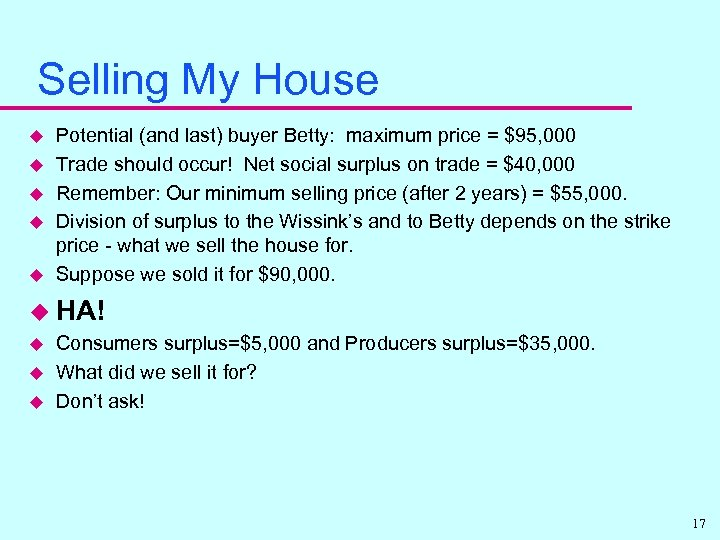Selling My House u u u Potential (and last) buyer Betty: maximum price = \$95, 000 Trade should occur! Net social surplus on trade = \$40, 000 Remember: Our minimum selling price (after 2 years) = \$55, 000. Division of surplus to the Wissink’s and to Betty depends on the strike price - what we sell the house for. Suppose we sold it for \$90, 000. u HA! u u u Consumers surplus=\$5, 000 and Producers surplus=\$35, 000. What did we sell it for? Don’t ask! 17